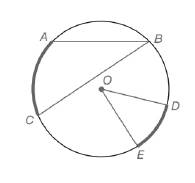Chapter 6.1, Problem 5E### Elementary Geometry for College St...

6th Edition
Daniel C. Alexander + 1 other
ISBN: 9781285195698

#### Solutions

Chapter
Section### Elementary Geometry for College St...

6th Edition
Daniel C. Alexander + 1 other
ISBN: 9781285195698
Textbook Problem
1 views

# For Exercises 1 to 8, use the figure provided.Exercises 1-8If m ∠ B = 28.3 ∘ ,  find m A C ⌢ .

To determine

To calculate:

The mAC, mB=28.3o by using the provided figure.

Explanation

Given:

The provided figure is given below:

Theorem used:

The measure of an inscribed angle of a circle is one-half the measure of its intercepted arc.

Calculation:

Given, mB=28.3o

Since, the measure of an inscribed angle of a circle is one-half the measure of its intercepted arc.

mB=12mAC28

### Still sussing out bartleby?

Check out a sample textbook solution.

See a sample solution

#### The Solution to Your Study Problems

Bartleby provides explanations to thousands of textbook problems written by our experts, many with advanced degrees!

Get Started